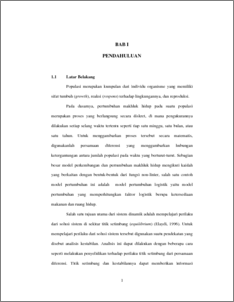# Stability Analysis of Non Linear Difference Equation

Uswatun, Indah and Sutimin, Sutimin and Kartono, Kartono (2009) Stability Analysis of Non Linear Difference Equation. Undergraduate thesis, Universitas Diponegoro.Preview
PDF
110Kb

## Abstract

Stability analysis of non-linear difference equation is conducted through a testing of the theorem which is done by investigating the equilibrium point . Stability analysis of difference equation in the previous theorem shown that if then the point is asymptotically stable, but if then the point is unstable, then if , the stability of equilibrium point has not been able to determine yet. In this final project, the stability of equilibrium point will be investigated in case which . The investigation conducted by calculating and factor so that stability of equilibrium point can be determined. Stability analysis can be conducted through Cobweb diagram. For difference logistic equation, stability of equilibrium point depend on values of parameter .

Item Type: Thesis (Undergraduate) stability, difference equation, equilibrium point, Cobweb diagram. Q Science > QA Mathematics Faculty of Science and Mathematics > Department of Mathematics 2927 INVALID USER 22 Dec 2009 14:58 22 Dec 2009 14:58

Repository Staff Only: item control page